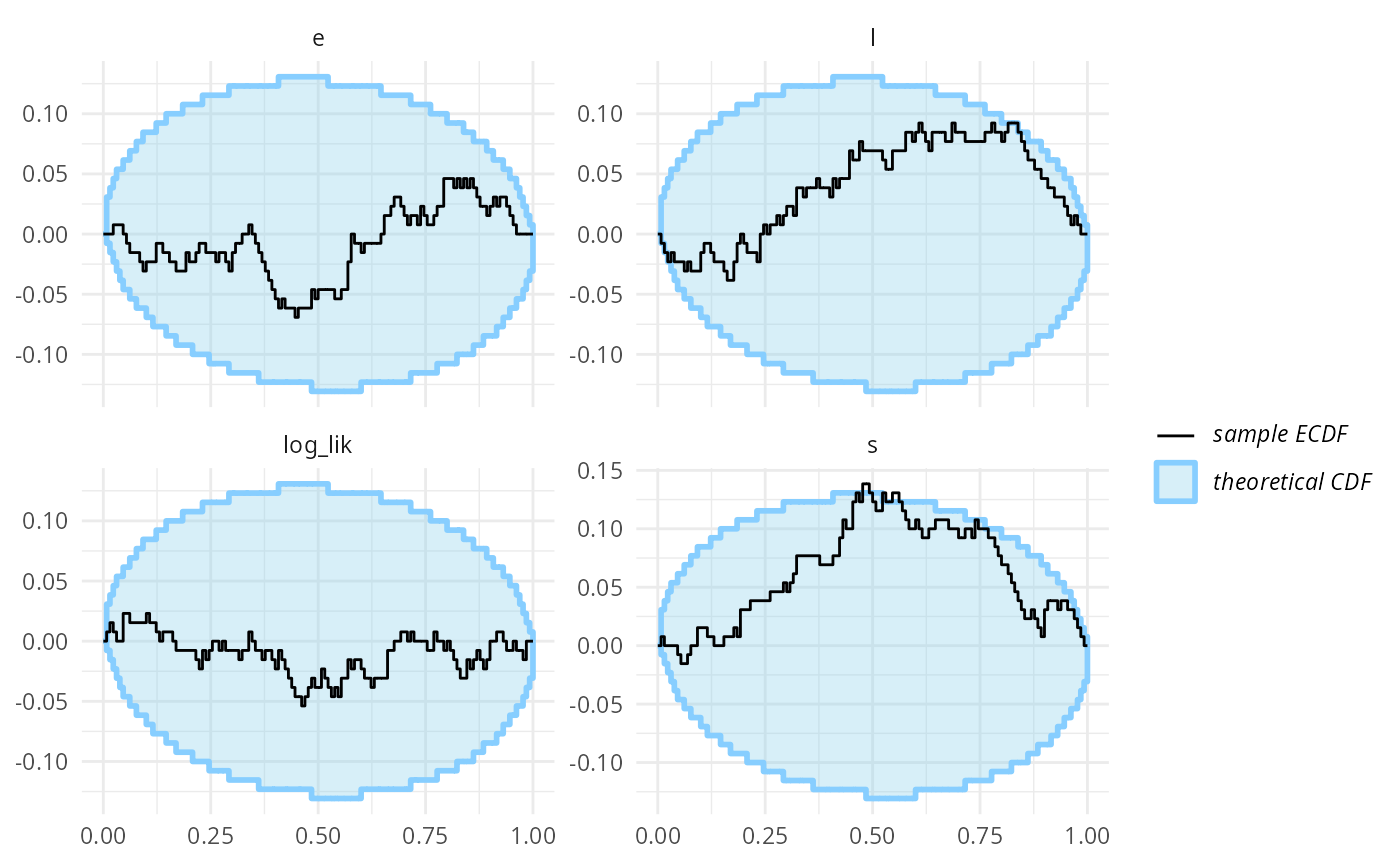SBC was primarily designed for continuous parameters, but can be used with models that have discrete parameters - whether the parameters are directly represented (e.g. in BUGS/JAGS) or marginalized out (as is usual in Stan).

## Stan version and debugging

library(SBC);
library(ggplot2)

use_cmdstanr <- getOption("SBC.vignettes_cmdstanr", TRUE) # Set to false to use rstan instead

if(use_cmdstanr) {
library(cmdstanr)
} else {
library(rstan)
rstan_options(auto_write = TRUE)
}

# Multiprocessing support
library(future)
plan(multisession)

# The fits are very fast and we fit just a few,
# so we force a minimum chunk size to reduce overhead of
# paralellization and decrease computation time.
options(SBC.min_chunk_size = 5)

# Setup caching of results
if(use_cmdstanr) {
cache_dir <- "./_discrete_vars_SBC_cache"
} else {
cache_dir <- "./_discrete_vars_rstan_SBC_cache"
}
cache_dir_jags <- "./_discrete_vars_SBC_cache"

if(!dir.exists(cache_dir)) {
dir.create(cache_dir)
}
if(!dir.exists(cache_dir_jags)) {
dir.create(cache_dir_jags)
}

We take the changepoint model from: https://mc-stan.org/docs/2_26/stan-users-guide/change-point-section.html

cat(readLines("stan/discrete_vars1.stan"), sep = "\n")
data {
real<lower=0> r_e;
real<lower=0> r_l;

int<lower=1> T;
int<lower=0> y[T];
}
transformed data {
real log_unif;
log_unif = -log(T);
}
parameters {
real<lower=0> e;
real<lower=0> l;
}
transformed parameters {
vector[T] lp;
lp = rep_vector(log_unif, T);
for (s in 1:T)
for (t in 1:T)
lp[s] = lp[s] + poisson_lpmf(y[t] | t < s ? e : l);
}
model {
e ~ exponential(r_e);
l ~ exponential(r_l);
target += log_sum_exp(lp);
}

generated quantities {
int<lower=1,upper=T> s;
s = categorical_logit_rng(lp);
}
if(use_cmdstanr) {
model_1 <- cmdstan_model("stan/discrete_vars1.stan")
backend_1 <- SBC_backend_cmdstan_sample(model_1)
} else {
model_1 <- stan_model("stan/discrete_vars1.stan")
backend_1 <- SBC_backend_rstan_sample(model_1)
}

Now, let’s generate data from the model.

generate_single_sim_1 <- function(T, r_e, r_l) {
e <- rexp(1, r_e)
l <- rexp(1, r_l)
s <- sample.int(T, size = 1)

y <- array(NA_real_, T)
for(t in 1:T) {
if(t <= s) {
rate <- e
} else {
rate <- l
}
y[t] <- rpois(1, rate)
}

list(
variables = list(
e = e, l = l, s = s
), generated = list(
T = T,
r_e = r_e,
r_l = r_l,
y = y
)
)
}

generator_1 <- SBC_generator_function(generate_single_sim_1, T = 5, r_e = 0.5, r_l = 0.1)
set.seed(85394672)
datasets_1 <- generate_datasets(generator_1, 30)

Additionally, we’ll add a generated quantity expressing the total log-likelihood of data given the fitted parameters. The expression within the generated_quantities() call is evaluated for both prior and posterior draws and included as another variable in SBC checks. It turns out this type of generated quantities can increase the sensitivity of the SBC against some issues in the model. See vignette("limits_of_SBC") for a more detailed discussion of this.

log_lik_gq <- generated_quantities(log_lik = sum(dpois(y, ifelse(1:T < s, e, l), log = TRUE)))

So finally, lets actually compute SBC:

results_1 <- compute_SBC(datasets_1, backend_1,
cache_mode = "results",
cache_location = file.path(cache_dir, "model1"),
gen_quants = log_lik_gq)
## Results loaded from cache file 'model1'
##  - 5 (17%) fits had at least one Rhat > 1.01. Largest Rhat was NA.
##  - 20 (67%) fits had tail ESS undefined or less than half of the maximum rank, potentially skewing
## the rank statistics. The lowest tail ESS was NA.
##  If the fits look good otherwise, increasing thin_ranks (via recompute_SBC_statistics)
## or number of posterior draws (by refitting) might help.
##  - 2 (7%) fits had divergent transitions. Maximum number of divergences was 3.
## Not all diagnostics are OK.
## You can learn more by inspecting $default_diagnostics,$backend_diagnostics
## and/or investigating $outputs/$messages/$warnings for detailed output from the backend. Here we also use the caching feature to avoid recomputing the fits when recompiling this vignette. In practice, caching is not necessary but is often useful. TODO the diagnostic failures are false positives, because Rhat and ESS don’t work very well for discrete parameters. We need to figure out how to handle this better. We can quickly note that the statistics for the s parameter are extreme - many ranks of 0 and extreme z-scores, including -Infinity. Seing just one or two such fits should be enough to convince us that there is something fundamentally wrong. dplyr::filter(results_1$stats, variable == "s") 
## # A tibble: 30 × 15
##    sim_id variable simulated_value  rank   z_score  mean median    sd   mad
##     <int> <chr>              <dbl> <dbl>     <dbl> <dbl>  <dbl> <dbl> <dbl>
##  1      1 s                      3   185    0.0182  2.97      3 1.61   2.97
##  2      2 s                      1    24   -1.90    2.02      2 0.537  0
##  3      3 s                      4   126   -1.37    4.67      5 0.489  0
##  4      4 s                      1    10   -2.85    2.86      3 0.651  0
##  5      5 s                      5   397    2.76    2.86      3 0.775  0
##  6      6 s                      2   271    0.0449  1.94      1 1.42   0
##  7      7 s                      3     0 -Inf       4         4 0      0
##  8      8 s                      2   129   -0.594   2.87      3 1.46   1.48
##  9      9 s                      2     0   -6.84    2.99      3 0.144  0
## 10     10 s                      2     3   -8.68    3.00      3 0.115  0
## # … with 20 more rows, and 6 more variables: q5 <dbl>, q95 <dbl>, rhat <dbl>,
## #   ess_bulk <dbl>, ess_tail <dbl>, max_rank <int>

Inspecting the statistics shows that quite often, the model is quite sure of the value of s while the simulated value is just one less.

Looking at the ecdf_diff plot we see that this seems to compromise heavily the inference for s, but the other parameters do not show such bad behaviour. Note that the log_lik generated quantity shows even starker failure than s, so it indeed poses a stricter check in this scenario.

plot_ecdf_diff(results_1)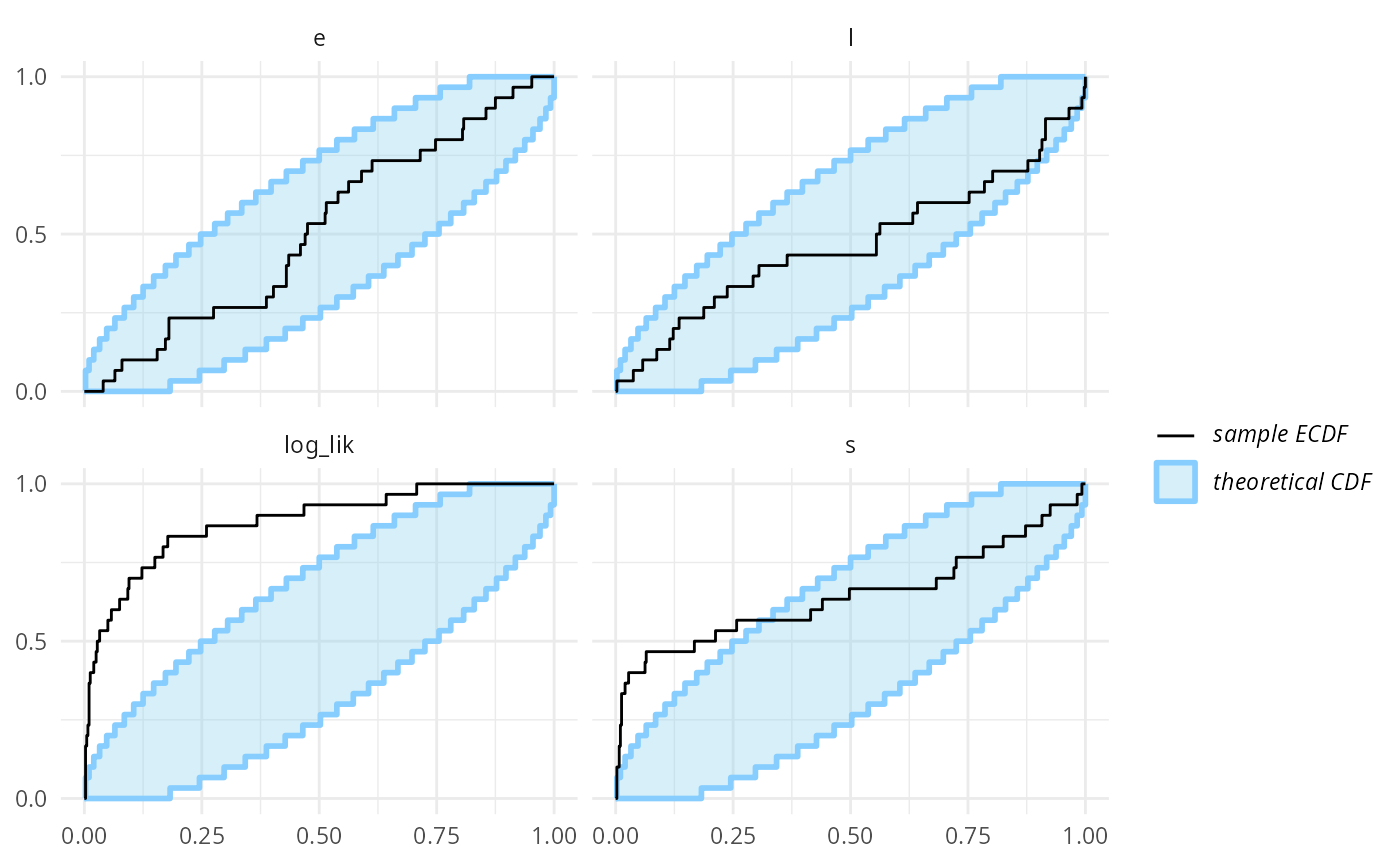plot_rank_hist(results_1)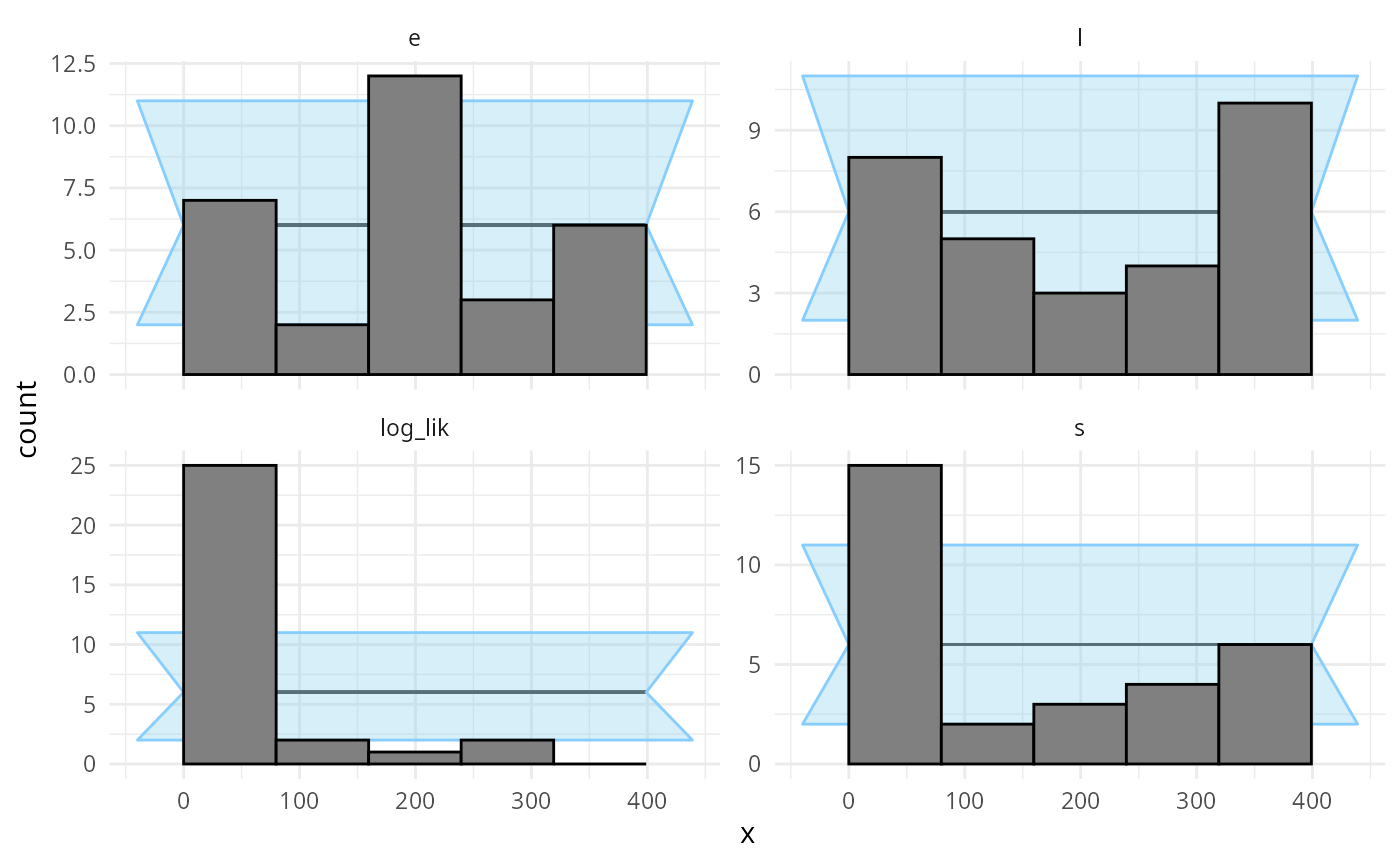An important note: you may wonder, how we got such a wiggly line for the s parameter - doesn’t it have just 5 possible values? Shouldn’t therefore the ECDF be one big staircase? In fact the package does a little trick to make discrete parameters comparable to continuous - the rank of a discrete parameter is chosen uniformly randomly across all possible ranks (i.e. posterior draws that have exactly equal value). This means that if the model is well behaved, ranks for the discrete parameter will be uniformly distributed across the whole range of possible ranks and we can use exactly the same diagnostics for a discrete parameter as we do for the continuous ones.

But back to the model - what happened? What is wrong with it? After some inspection, you may notice that the simulator does not match the model - the model takes the early rate (e) for points t < s while the simulator takes e for points t <= s, so there is effectively a shift by one time point between the simulator and the model. So let’s assume that we beleive that the Stan model is in fact right. We therefore updated the simulator to match the model:

generate_single_sim_2 <- function(T, r_e, r_l) {
e <- rexp(1, r_e)
l <- rexp(1, r_l)
s <- sample.int(T, size = 1)

y <- array(NA_real_, T)
for(t in 1:T) {
if(t < s) { ### <--- Only change here
rate <- e
} else {
rate <- l
}
y[t] <- rpois(1, rate)
}

list(
variables = list(
e = e, l = l, s = s
), generated = list(
T = T,
r_e = r_e,
r_l = r_l,
y = y
)
)
}

generator_2 <- SBC_generator_function(generate_single_sim_2, T = 5, r_e = 0.5, r_l = 0.1)

And we can recompute:

set.seed(5846502)
datasets_2 <- generate_datasets(generator_2, 30)
results_2 <- compute_SBC(datasets_2, backend_1,
gen_quants = log_lik_gq,
cache_mode = "results",
cache_location = file.path(cache_dir, "model2"))
## Results loaded from cache file 'model2'
##  - 8 (27%) fits had at least one Rhat > 1.01. Largest Rhat was NA.
##  - 24 (80%) fits had tail ESS undefined or less than half of the maximum rank, potentially skewing
## the rank statistics. The lowest tail ESS was NA.
##  If the fits look good otherwise, increasing thin_ranks (via recompute_SBC_statistics)
## or number of posterior draws (by refitting) might help.
##  - 2 (7%) fits had divergent transitions. Maximum number of divergences was 2.
## Not all diagnostics are OK.
## You can learn more by inspecting $default_diagnostics,$backend_diagnostics
## and/or investigating $outputs/$messages/$warnings for detailed output from the backend. plot_rank_hist(results_2)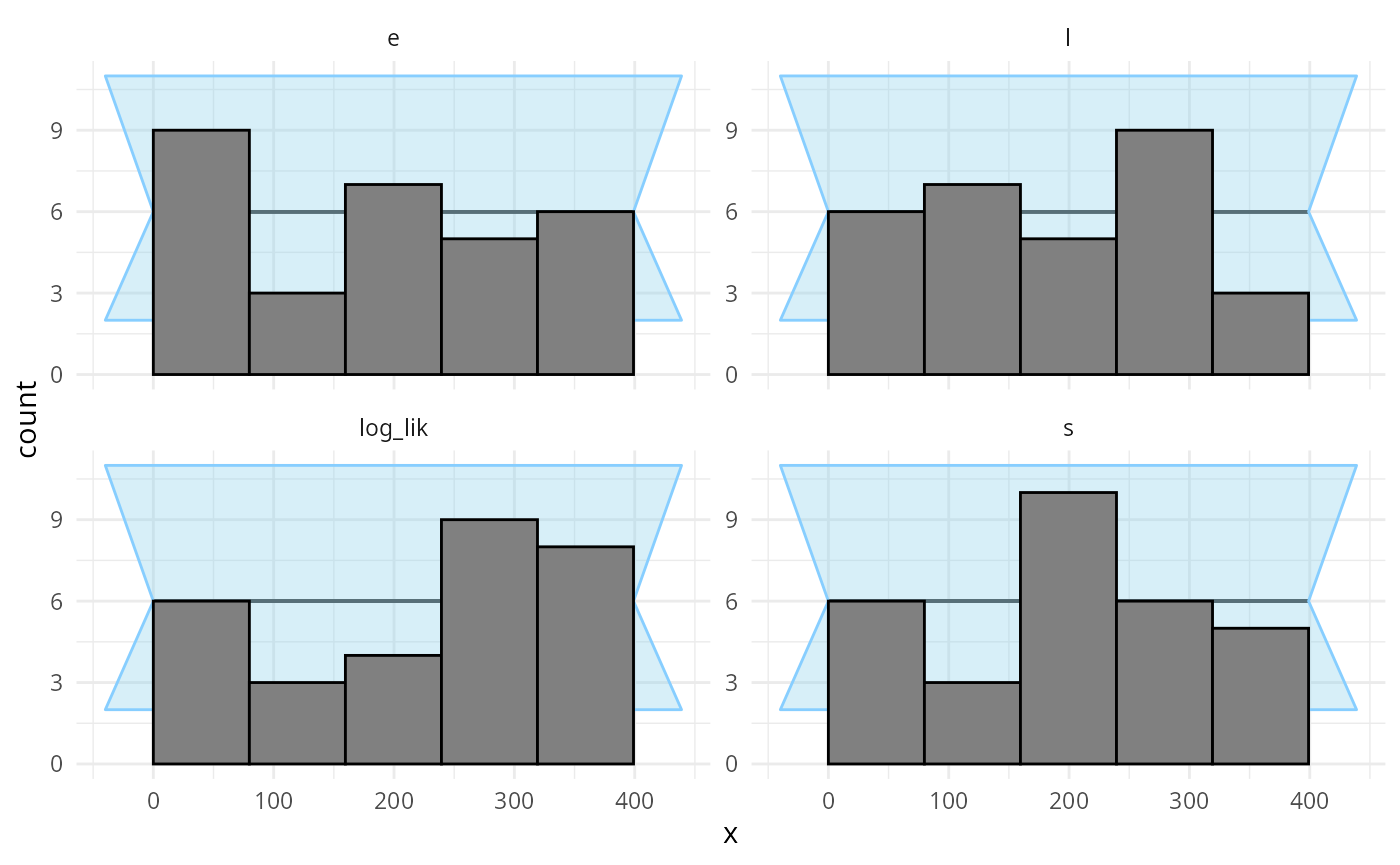plot_ecdf_diff(results_2)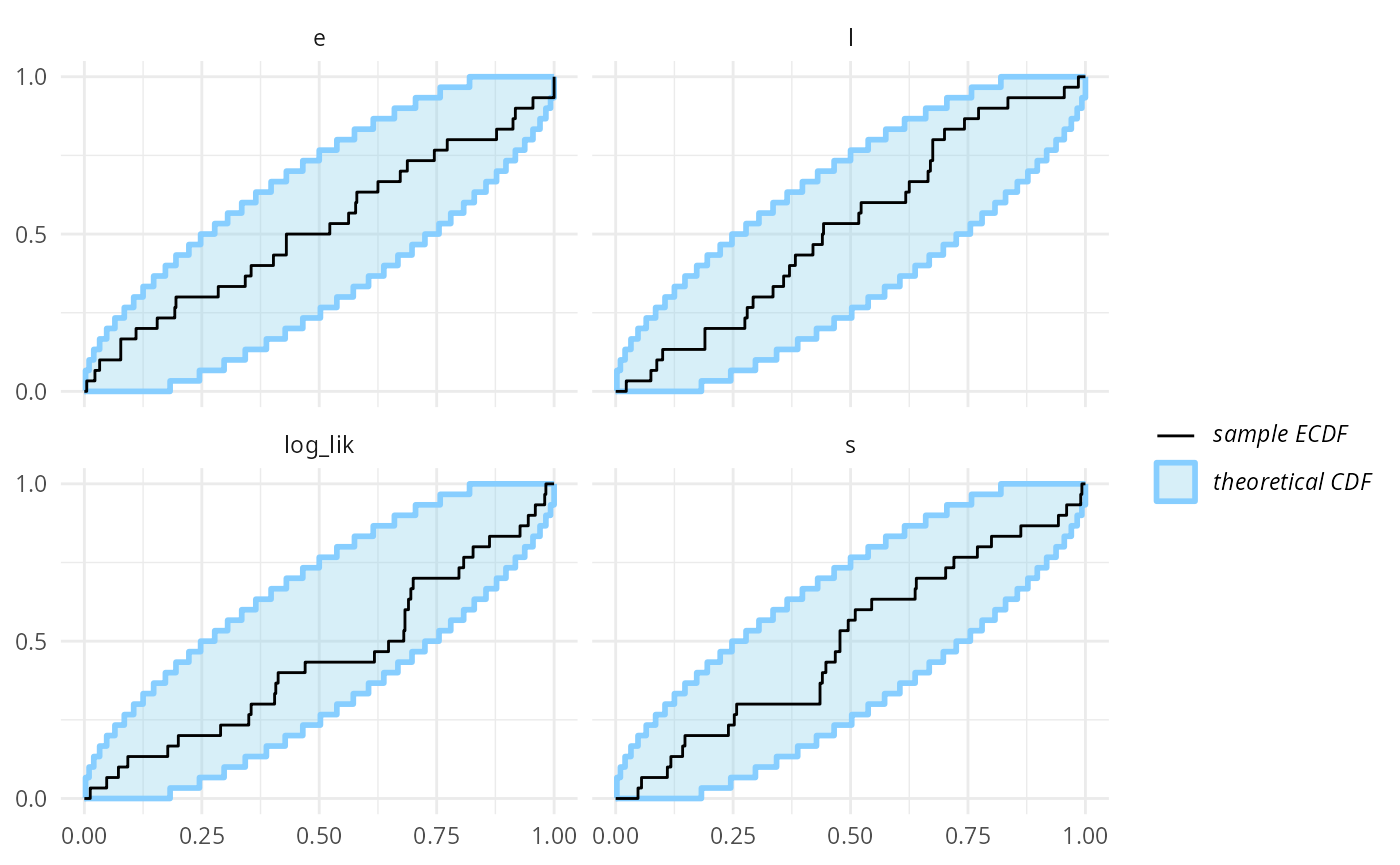Looks good, so let us add some more simulations to make sure the model behaves well. set.seed(54321488) datasets_2_more <- generate_datasets(generator_2, 100) results_2_more <- compute_SBC(datasets_2_more, backend_1, gen_quants = log_lik_gq, cache_mode = "results", cache_location = file.path(cache_dir, "model3")) ## Results loaded from cache file 'model3' ## - 15 (15%) fits had at least one Rhat > 1.01. Largest Rhat was NA. ## - 73 (73%) fits had tail ESS undefined or less than half of the maximum rank, potentially skewing ## the rank statistics. The lowest tail ESS was NA. ## If the fits look good otherwise, increasing thin_ranks (via recompute_SBC_statistics) ## or number of posterior draws (by refitting) might help. ## - 7 (7%) fits had divergent transitions. Maximum number of divergences was 20. ## Not all diagnostics are OK. ## You can learn more by inspecting$default_diagnostics, $backend_diagnostics ## and/or investigating$outputs/$messages/$warnings for detailed output from the backend.
results_2_all <- bind_results(results_2, results_2_more)
plot_rank_hist(results_2_all)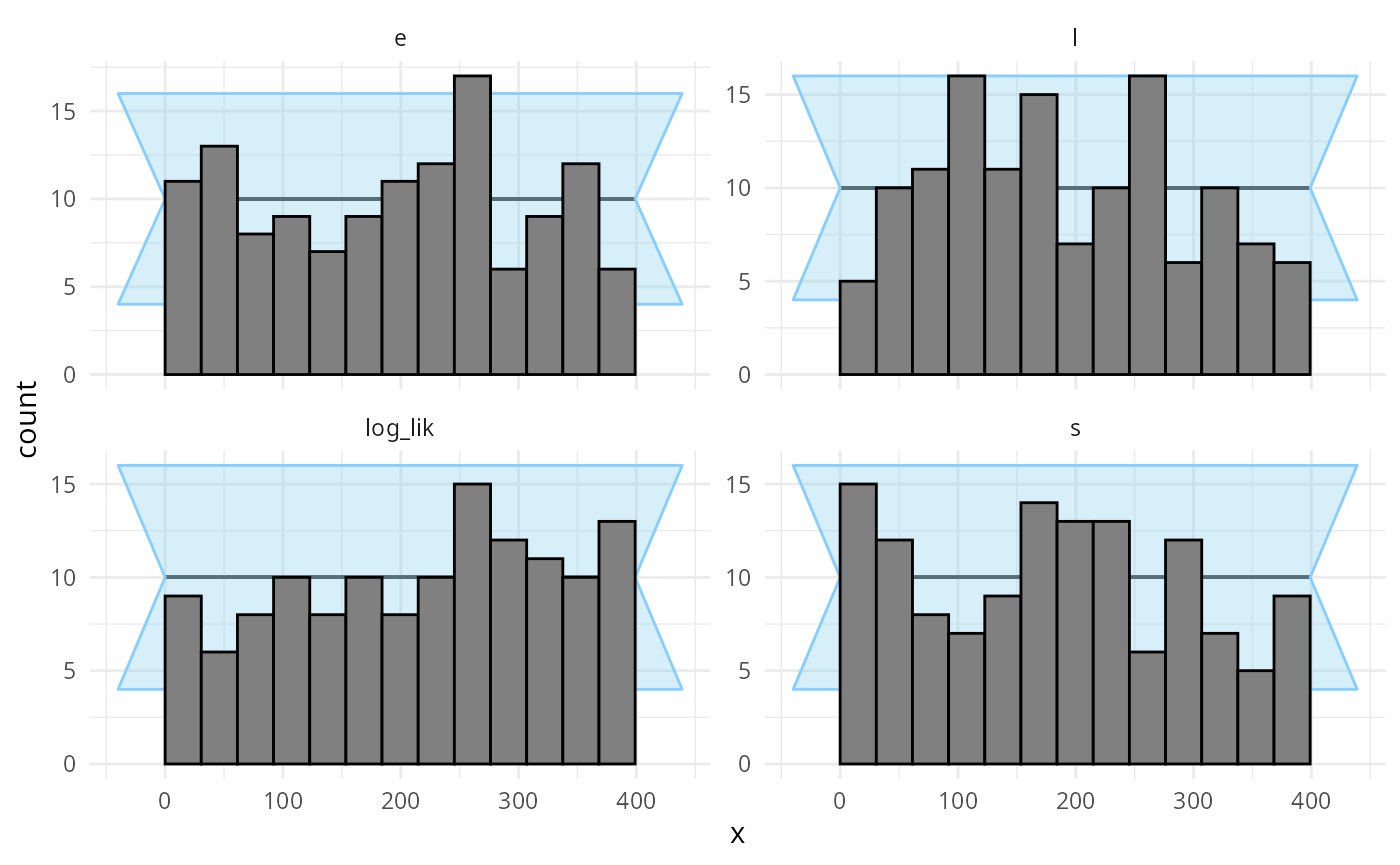plot_ecdf_diff(results_2_all)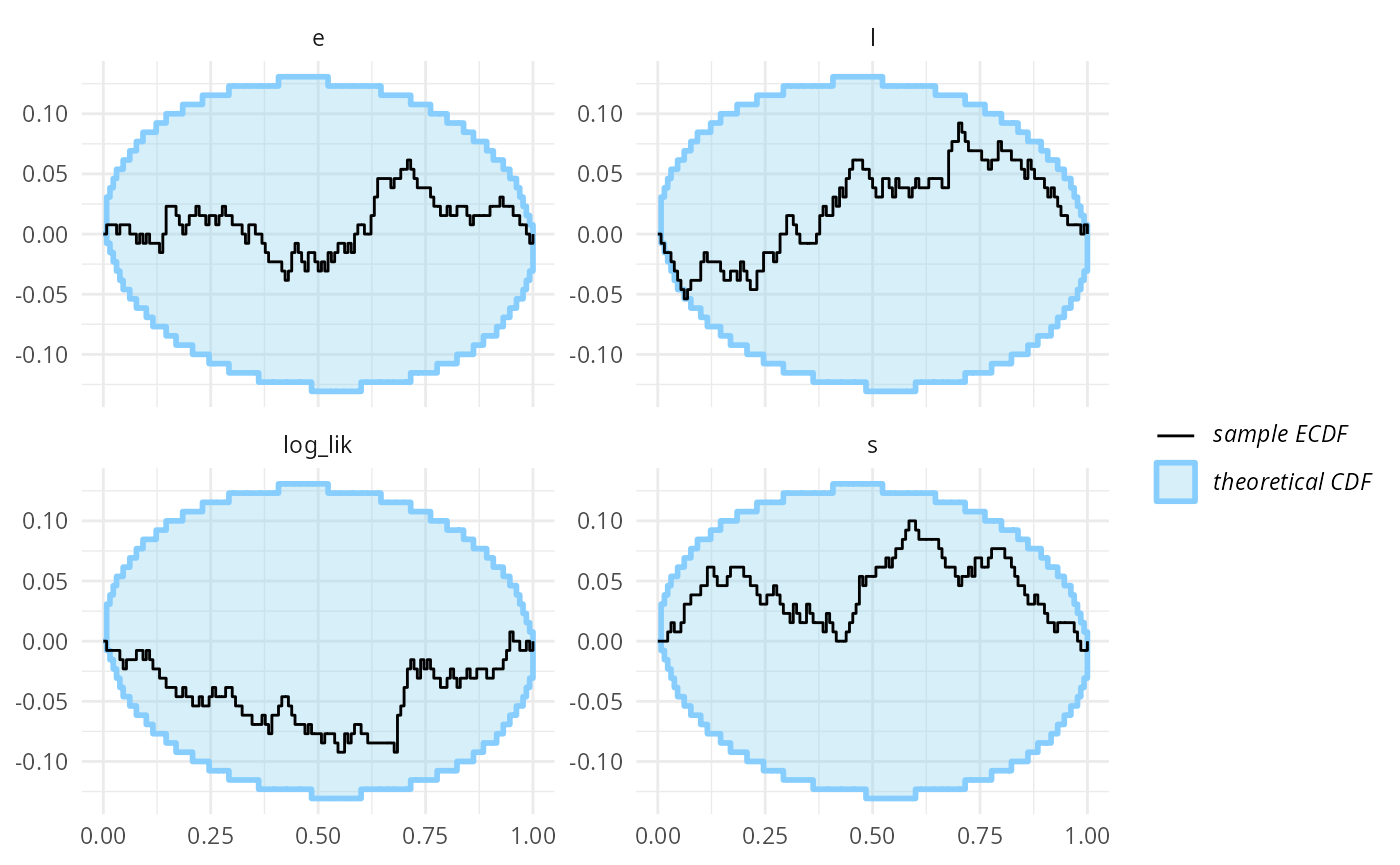Now - as far as this amount of SBC steps can see, the model is good and we get good behaviour for both the continuous and the discrete parameters and the log_lik generated quantity. Hooray!

## JAGS version

We can now write the same model in JAGS. This becomes a bit easier as JAGS lets us represent discrete parameters directly:

cat(readLines("other_models/changepoint.jags"), sep = "\n")
data {
for(i in 1:T) {
prior_s[i] = 1.0/T
}
}

model {
e ~ dexp(r_e);
l ~ dexp(r_l);
s ~ dcat(prior_s)
for(i in 1:T) {
y[i] ~ dpois(ifelse(i < s, e, l))
}
}

We will use the rjags package, let us verify it is installed correctly.

library(rjags)
## Loading required package: coda
## Linked to JAGS 4.3.1
## Loaded modules: basemod,bugs

We will also default to relatively large number of samples as we can expect some autocorrelation in the Gibbs sampler.

backend_jags <- SBC_backend_rjags("other_models/changepoint.jags",
variable.names = c("e","l","s"),
n.iter = 10000,
n.burnin = 1000,
n.chains = 4,
thin = 10)

Running SBC with all the corrected datasets from before (rJAGS accepts input data in exactly the same format as Stan, so we can reuse the datasets without any change):

datasets_2_all <- bind_datasets(datasets_2, datasets_2_more)
results_jags <- compute_SBC(datasets_2_all, backend_jags,
gen_quants = log_lik_gq,
cache_mode = "results",
cache_location = file.path(cache_dir_jags, "rjags"))
## Results loaded from cache file 'rjags'
##  - 21 (16%) fits had at least one Rhat > 1.01. Largest Rhat was NA.
##  - 95 (73%) fits had tail ESS undefined or less than half of the maximum rank, potentially skewing
## the rank statistics. The lowest tail ESS was NA.
##  If the fits look good otherwise, increasing thin_ranks (via recompute_SBC_statistics)
## or number of posterior draws (by refitting) might help.
## Not all diagnostics are OK.
## You can learn more by inspecting $default_diagnostics,$backend_diagnostics
## and/or investigating $outputs/$messages/$warnings for detailed output from the backend. Similarly to the case above, the Rhat and ESS warnings are false positives due to the s parameter, which we need to handle better. The rank plots show no problems. plot_rank_hist(results_jags)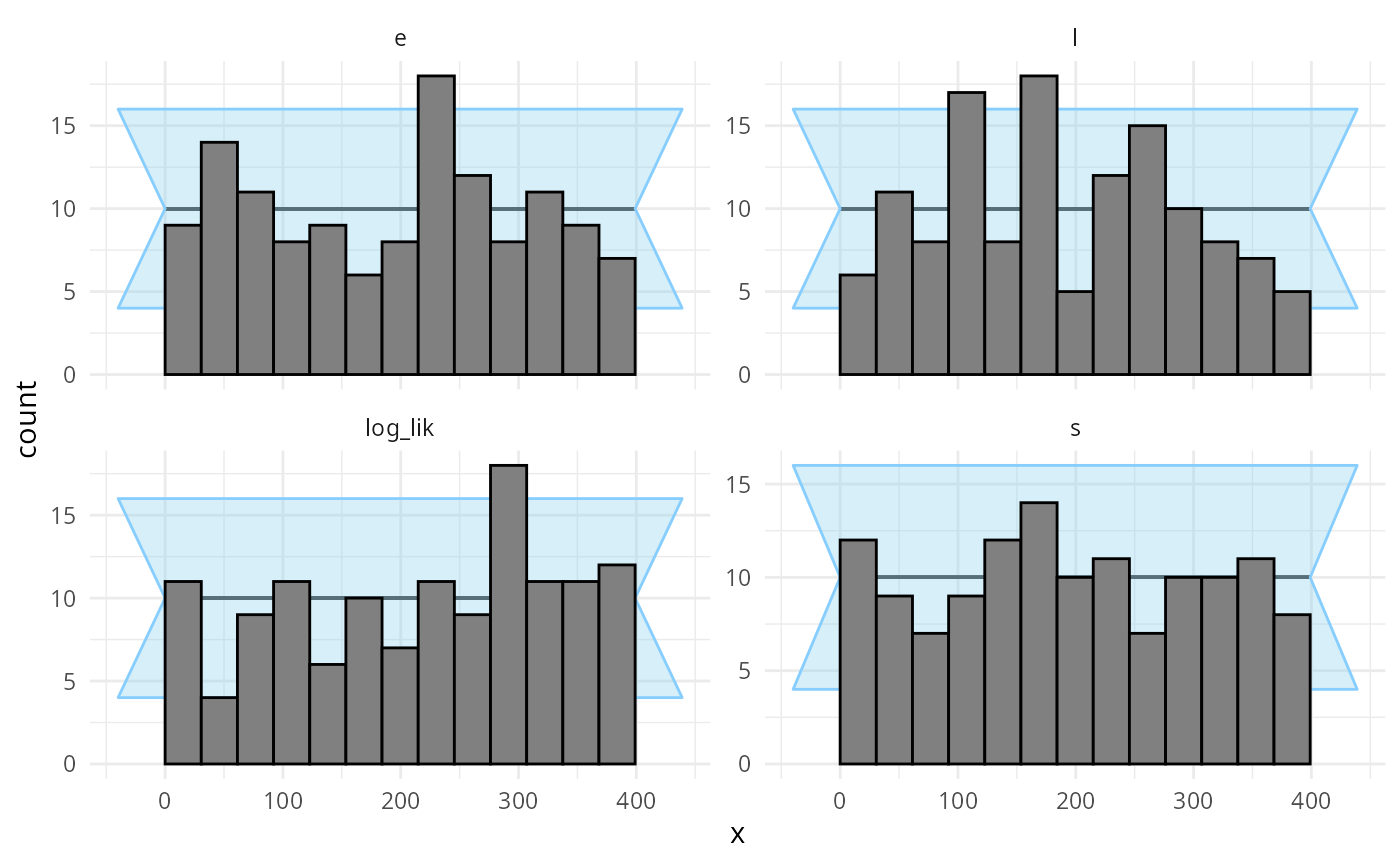plot_ecdf_diff(results_jags)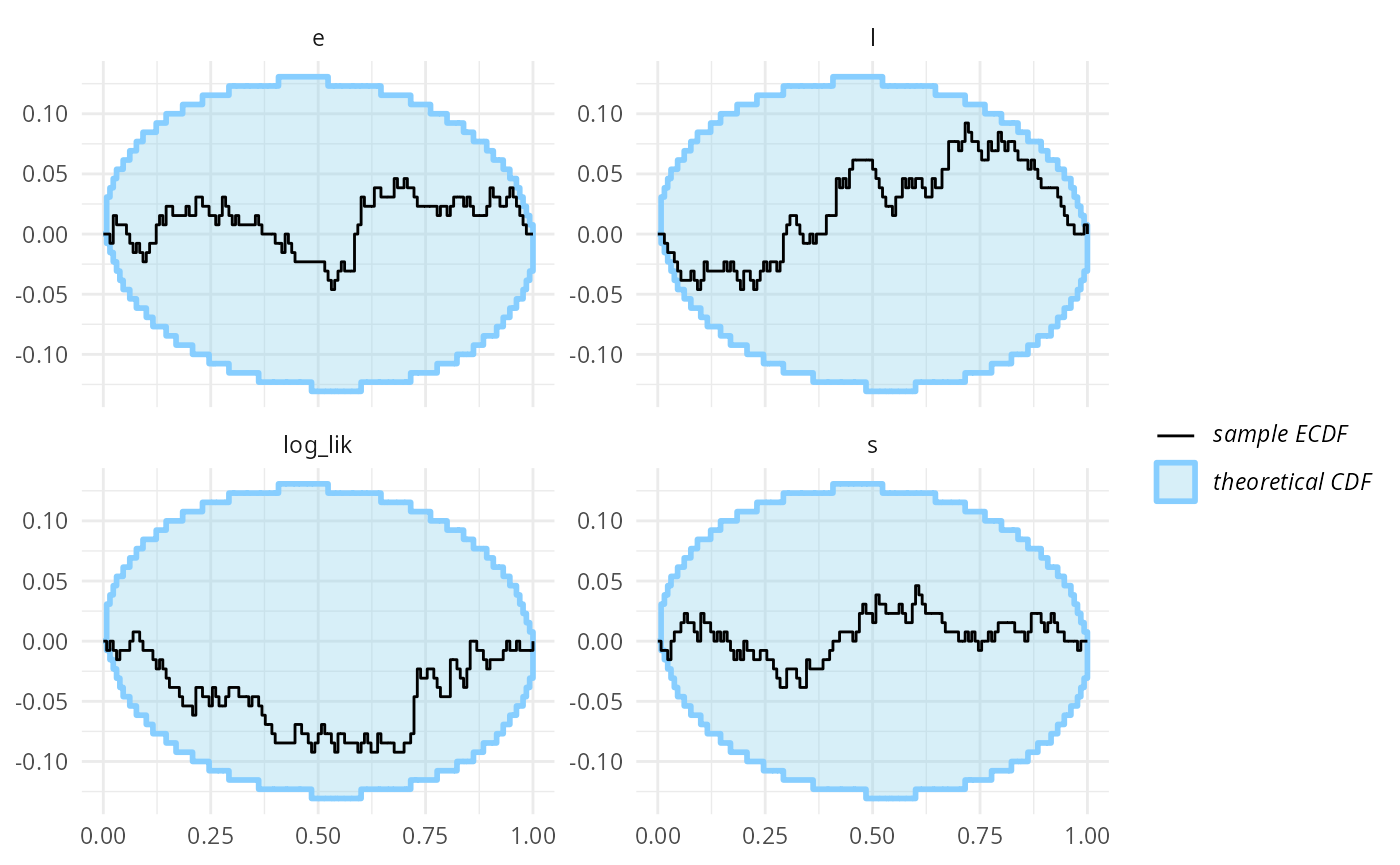As an exercise, we can also write the marginalized version of the model in JAGS. In some cases, marginalization improves performance even for JAGS models, however, for this model it is actually not an improvement, presumably because the model is very simple. cat(readLines("other_models/changepoint_marginalized.jags"), sep = "\n") data { for(i in 1:T) { prior_unif[i] = -log(T) } # Using the zeroes crossing trick to compute the likelihood # See e.g. https://667-per-cm.net/2014/02/17/the-zero-crossings-trick-for-jags-finding-roots-stochastically/ z = 0 } model { e ~ dexp(r_e); l ~ dexp(r_l); # Prepare the zero trick z ~ dpois(z_mean) # Compute the likelihood # The lp is a matrix to avoid having to redefine nodes lp[1, 1:T] = prior_unif for (s in 1:T) { for (t in 1:T) { lp[1 + t, s] = lp[t, s] + log(ifelse(t < s, e, l)) * y[t] - ifelse(t < s, e, l) } p[s] = exp(lp[T + 1, s]) } # log-sum-exp to compute the log likelihood in a numerically stable way m = max(lp[T + 1, ]) sum_exp_rest = 0 for(t in 1:T) { sum_exp_rest[1 + t] = sum_exp_rest[t] + exp(lp[T + 1, s] - m) } lp_total = m + log(sum_exp_rest[T + 1]) # We have the likelihood now add it to z_mean for the zeros trick z_mean = -lp_total + 10000 s ~ dcat(p) } The code got quite a bit more complex, se let’s check if we didn’t mess up the rewrite - first we build a backend with this new representation: backend_jags_marginalized <- SBC_backend_rjags("other_models/changepoint_marginalized.jags", variable.names = c("e","l","s"), n.iter = 10000, n.burnin = 1000, n.chains = 4, thin = 10) Then we run the actual SBC: results_jags_marginalized <- compute_SBC(datasets_2_all, backend_jags_marginalized, gen_quants = log_lik_gq, cache_mode = "results", cache_location = file.path(cache_dir_jags, "rjags_marginalized")) ## Results loaded from cache file 'rjags_marginalized' ## - 24 (18%) fits had at least one Rhat > 1.01. Largest Rhat was NA. ## - 89 (68%) fits had tail ESS undefined or less than half of the maximum rank, potentially skewing ## the rank statistics. The lowest tail ESS was NA. ## If the fits look good otherwise, increasing thin_ranks (via recompute_SBC_statistics) ## or number of posterior draws (by refitting) might help. ## Not all diagnostics are OK. ## You can learn more by inspecting$default_diagnostics, $backend_diagnostics ## and/or investigating$outputs/$messages/$warnings for detailed output from the backend.

And the ranks plots look good, so we indeed probably did succeed in correctly marginalizing the s parameter!

plot_rank_hist(results_jags_marginalized)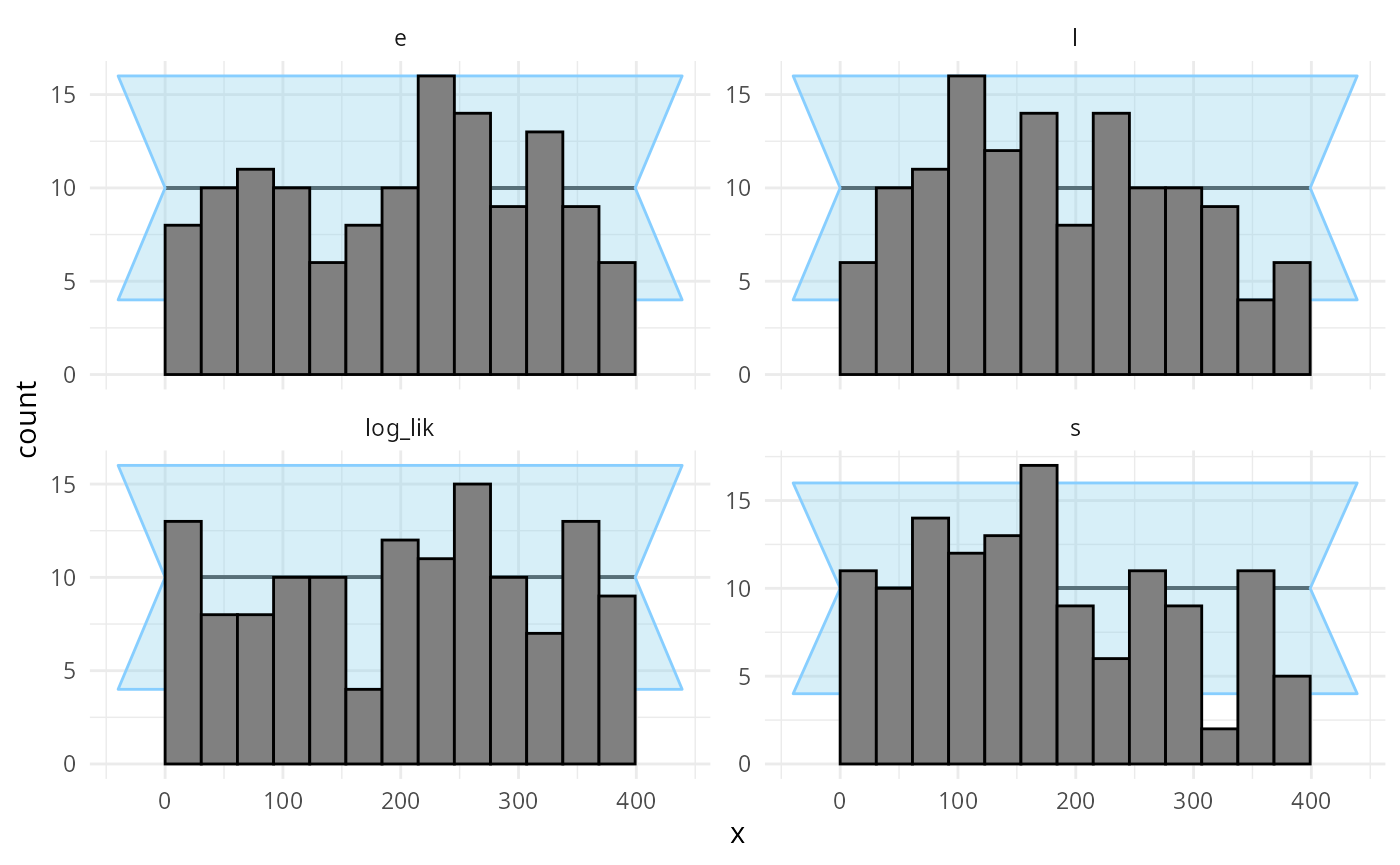plot_ecdf_diff(results_jags_marginalized)